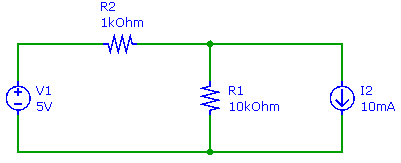## Node-voltage analysis Assignment Help

Assignment Help: >> Electrical Engineering - Node-voltage analysis

Node-voltage analysis

The Node voltage analysis is a systematic technique for finding the voltage at all nodes in an electrical circuit. We describe the steps of the fundamental procedure for the illustration shown in the figure below. The node voltage analysis (that is, Nodal analysis) is another type of circuit or network analysis method that will solve almost any linear circuit. In a manner, this technique entirely analogous to mesh analysis technique, writes KCL equations rather than KVL equations, and solves them concurrently.Assign a reference node Assign node voltages Calculate currents Compute current I flowing via the battery using Node voltage Identify all nodes in the circuit Identify unknown node voltages Solve for unknown node voltages Write KCL equation